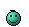# MATH TIME!

Discussion in 'User Topics' started by Mr.Lame, Jan 9, 2018.

•Like x 1
1. ### Mr.LameVeteran

Blogs:
28
Joined:
Dec 7, 2009
Posts:
1,540
628
@MarkFL l I don't even knnnooowww

#### Attached Files:

• ###### Screen Shot 2018-01-21 at 5.32.25 PM.png
File size:
21.4 KB
Views:
1
•Like x 1
•Like x 1
2. ### Mr.LameVeteran

Blogs:
28
Joined:
Dec 7, 2009
Posts:
1,540
628
@MarkFL Hey mark I was wondering if you could pass me 2-3 difference of cube questions, nothing too wild, something like a^3+8/a^2-4 Thank you3. ### Mr.LameVeteran

Blogs:
28
Joined:
Dec 7, 2009
Posts:
1,540
628
@MarkFL OH and, how does the q move to the six instead of staying to the 3^2

5q^2/6 * 2^2/3^2q^4

which ends up 2^2*5 / 3^2*6q^2

4. When I'm in for the night, I will more fully answer, but just recall that multiplication is commutative, which means essentially that:

ab = ba

But, I will show you tonight how I would completely simplify that rational expression as well as give you some difference of cubes problems.•Like x 1
5. ### Mr.LameVeteran

Blogs:
28
Joined:
Dec 7, 2009
Posts:
1,540
628
I kinda cheated so I could see how it was done when I got stuck, but because it doesn't have actual descriptions trying to comprehend it is like learning a new language lol you can see how it's used and you can copy the words but no idea what you're saying6. ### Mr.LameVeteran

Blogs:
28
Joined:
Dec 7, 2009
Posts:
1,540
628
How do we break the 6 apart though? can I do with if it were an eight? Say that instead of three it was a 4?

7. Any number can be written as the product of its prime factors...for example:

6 = 2*3
8 = 2*2*2 = 2^3

•Like x 1
8. ### Mr.LameVeteran

Blogs:
28
Joined:
Dec 7, 2009
Posts:
1,540
628
Interesting, so we just add the exponent and remove the like term to simplify! O.O

9. Yes, add exponents, and then divide you and factors common to numerator and denominator. We can do the same thing with pure numbers...for example:

12/60 = (2^2*3)/(2^2*3*5) = 1/5

•Winner x 1
10. ### Mr.LameVeteran

Blogs:
28
Joined:
Dec 7, 2009
Posts:
1,540
628
Sweeeeet, okay I feel comfortable with this!•Winner x 1
11. ### Mr.LameVeteran

Blogs:
28
Joined:
Dec 7, 2009
Posts:
1,540
628
OH and you'd be proud I did 4(x-2)^2+15=0 Correctly!!! Even remembered to put the imaginary number (i) in front of the root!

•Winner x 1
12. ### Mr.LameVeteran

Blogs:
28
Joined:
Dec 7, 2009
Posts:
1,540
628
@MarkFL How does one complete the square?

X^2+7x-2=0?

13. Okay first you want to group the two terms containing x:

(x^2 + 7x) - 2 = 0

Now, inside the brackets, you want to look at the coefficient of the term with just x...it is 7. You want to divide that by 2 and then square that...(7/2)^2 and you want to add this inside the brackets, and subtract it on the outside so that the net result is that you are adding zero to the left side, and so it is the same value:

(x^2 + 7x + (7/2)^2) - 2 - (7/2)^2 = 0

Now what you have in the brackets is a perfect square, and go ahead and square the term you subtracted:

(x + 7/2)^2 - 2 - 49/4 = 0

Combine the two terms outside the brackets:

(x + 7/2)^2 - 8/4 - 49/4 = 0

(x + 7/2)^2 - 57/4 = 0

(x + 7/2)^2 = 57/4

Take the square root of both sides:

x + 7/2 = ±√(57)/2

Subtract 7/2 from both sides:

x = -7/2 ± √(57)/2 = (-7 ± √(57))/2

14. ### Mr.LameVeteran

Blogs:
28
Joined:
Dec 7, 2009
Posts:
1,540
628
@MarkFL

A= H/2(b1+b2) solve for b1

Blogs:
28
Joined:
Dec 7, 2009
Posts:
1,540